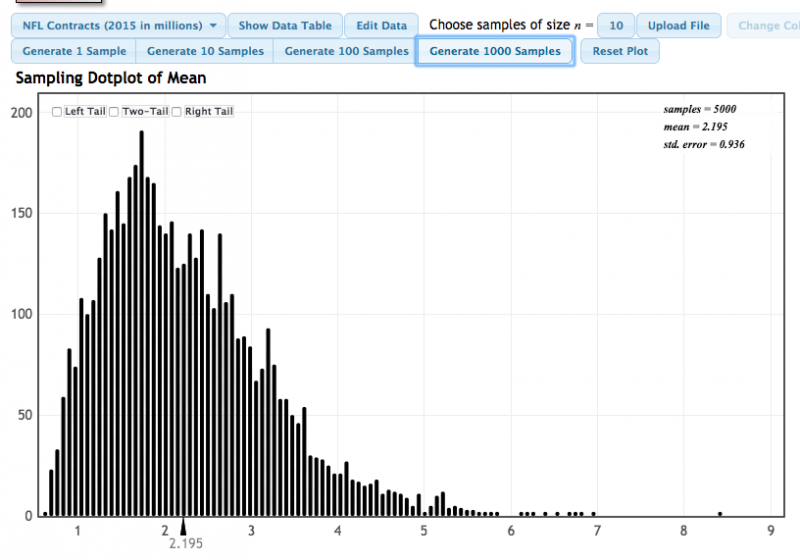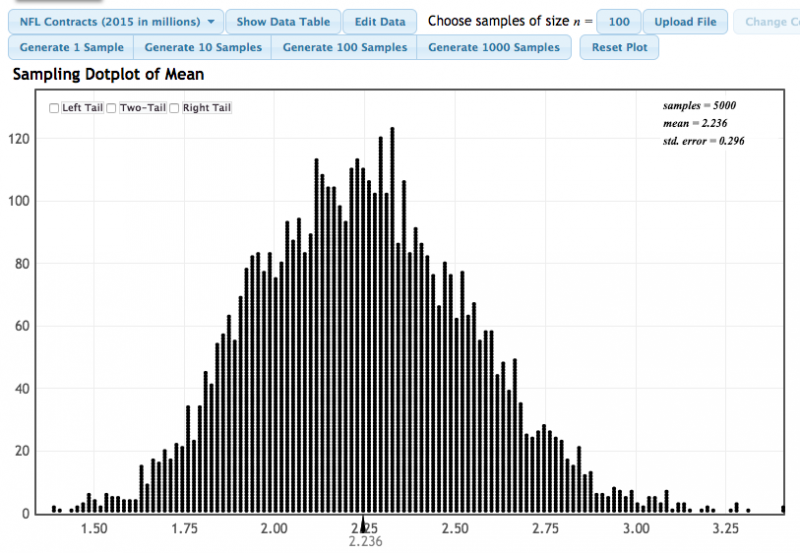# 4.1 - Sampling Distributions

4.1 - Sampling Distributions

Sample statistics are random variables because they vary from sample to sample. As a result, sample statistics have a distribution called the sampling distribution. The video below demonstrates the construction of a sampling distribution for a known population proportion using StatKey (http://www.lock5stat.com/StatKey/index.html). StatKey is a free online application that we will be using throughout the course.

An important aspect of a sampling distribution is the standard error (SE). The standard error is the standard deviation of a sampling distribution.  For a single categorical variable this may be referred to as the standard error of the proportion. For a single quantitative variable this may be referred to as the standard error of the mean. If a sampling distribution is constructed using data from a population, the mean of the sampling distribution will be approximately equal to the population parameter.

Sampling Distribution
Distribution of sample statistics with a mean approximately equal to the mean in the original distribution and a standard deviation known as the standard error
Standard Error
Standard deviation of a sampling distribution
Using StatKey to Construct a Sampling Distribution Given a Known Population Proportion

Note that this method of constructing a sampling distribution requires that we have population data. In most cases we do not know all of the population values. If we did, then we wouldn't need to construct a confidence interval to estimate the population parameter! In those cases we can use bootstrapping methods which you will see in the next section.

As you look through the following examples, note that when the sample size is large the sampling distribution is approximately symmetrical and centered at the population parameter.

# 4.1.1 - StatKey Examples

4.1.1 - StatKey Examples

The process of constructing a sampling distribution from a known population is the same for all types of parameters (i.e., one group proportion, one group mean, difference in two proportions, difference in two means, simple linear regression slope, and correlation). In each case we take a simple random sample of $$n$$ from the population without replacement, record the sample statistic of interest, return those observations back into the population, and repeat many times. That distribution of sample statistics is known as the sampling distribution. If the sample size is large, the sampling distribution will be approximately normally with a mean equal to the population parameter.

The following pages include examples of using StatKey to construct sampling distributions for one mean and one proportion.

# 4.1.1.1 - NFL Salaries (One Mean)

4.1.1.1 - NFL Salaries (One Mean)

In this video a sampling distribution is constructed using the "NFL Contracts (2015 in Millions)" dataset that is built into the sampling distribution for a mean feature in StatKey. This dataset includes the salaries of all 2,099 NFL players in 2015 as of the start of that season. We'll construct a sampling distribution given $$n = 5$$.

# 4.1.1.2 - Coin Flipping (One Proportion)

4.1.1.2 - Coin Flipping (One Proportion)

We are conducting an experiment in which we are flipping a fair coin 5 times and counting how many times we flip heads. Whether or not the coin lands on heads is a categorical variable with a probability of 0.50. Let's use StatKey to construct a distribution of sample proportions that we could use to determine the probability of any of the possible combinations of successes and failures.

# 4.1.2 - Copying Data into StatKey

4.1.2 - Copying Data into StatKey

StatKey has a number of built-in datasets. These are great for practicing or for demonstration purposes. In real life, you will have your own datasets that are not built in. For a categorical variable, we can change the population proportion as we did in the example on Lesson 4.1.1.2. For a quantitative variable, we need to copy all of our data into StatKey. The video below walks through an example of copying data from Excel into StatKey.  The same procedures can be used to copy data from Minitab, or any other program, into StatKey.

This example uses data from

PATownsPop.xls

# 4.1.3 - Impact of Sample Size

4.1.3 - Impact of Sample Size

There is an inverse relationship between sample size and standard error.  In other words, as the sample size increases, the variability of sampling distribution decreases.

Also, as the sample size increases the shape of the sampling distribution becomes more similar to a normal distribution regardless of the shape of the population.

Example: Mean NFL Salary

The built-in dataset "NFL Contracts (2015 in millions)" was used to construct the two sampling distributions below. In the first, a sample size of 10 was used. In the second, a sample size of 100 was used.

#### Sample size of 10:#### Sample size of 100:With a sample size of 10, the standard error of the mean was 0.936. With a sample size of 100 the standard error of the mean was 0.296. When the sample size increased the standard error decreased.

Also know that the population was strongly skewed to the right. With the smaller sample size, the sampling distribution was also skewed to the right, though not as strongly skewed as the population. With the larger sample size, the sampling distribution was approximately normal.

## Example: Proportion of College Graduates

The built-in dataset "College Graduates" was used to construct the two sampling distributions below. In the first, a sample size of 10 was used. In the second, a sample size of 100 was used.

#### Sample size of 10:#### Sample size of 100:With a sample size of 10, the standard error of the mean was 0.143. With a sample size of 100 the standard error of the mean was 0.044. As the sample size increased the standard error decreased.

Also note how the shape of the sampling distribution changed. With the smaller sample size there were large gaps between each possible sample proportion. When the sample size increased, the gaps between the possible sampling proportions decreased. With the larger sampling size the sampling distribution approximates a normal distribution.

  Link ↥ Has Tooltip/Popover Toggleable Visibility